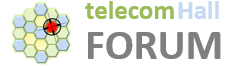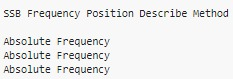# Calculate SSB frequency position in LTE TDD basead in NARFCN

Hi Experts,
How can I calculate SSB frequency position in LTE TDD basead in NARFCN?We are using Absolute Frequency in SSB Frequency Position Describe Method.

Sorry but why do you need SSB frequency for LTE?

In LTE position is fixed.

OK.
Based in my NARFCN, how to calculate the SSB Position?
DL NARFCN is 633334 and SSB position is 633322.
How can I calculate it (633322)?

NR ARFCN.
ARFCN for GSCN.
GSCN channel number.

But we don’t use GSCN.

In Huawei:
When SsbDescMethod is set to SSB_DESC_TYPE_GSCN (Global Synchronization Channel Number), this parameter indicates the global synchronization channel number corresponding to the cell SSB. When SsbDescMethod is set to SSB_DESC_TYPE_NARFCN (Absolute Frequency), this parameter indicates the absolute frequency number corresponding to the cell SSB.

It’s set to SSB_DESC_TYPE_NARFCN.

To calculate the “5G NR GSCN (SSB FREQUENCY)” is easy when I use SSB_DESC_TYPE_GSCN (i.e. using this calculator Telecom Calculators) but the problem is I´m not using it, it set to SSB_DESC_TYPE_GSCN

In both cases it is about Resource Element 0 center frequency of the PRB10 of the SSB.

This is another place you can do the calculation: Telecom Calculators (RF and Wireless Converters and Calculators)

What’s the value of your ssbGSCN parameter used under NRCellDU?
Is it 7846🤔 or different?
Just to confirm.

We don’t use GSCN (SSB Frequency Position Describe Method = Absolute Frequency). We are using NSA. TDD.

Hello Experts,

I found a solution, then sharing it here.According to a colleague here in the forum, the SSB Frequency can be any value as long as it is allocated within the range used.

There’s no problem that DL ARFCN (middle of spectrum) has different value than SSB frequency.

There is no calculation for SSB Frequency position in NSA!

The important is: If the SSB is beyond this range, the cell cannot be setup or work properly.

1 Like

Experts,